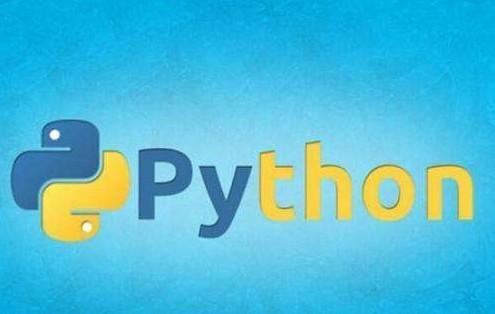# 内存占用如何python3中的代码解决？```import sys

variable = 30
print(sys.getsizeof(variable)) # 24```

Python是一个解释器，我们可以在任何时候扩展我们的类，例如，添加一个新的字段:

```d1 = DataItem("Alex", 42, "-")
print ("get_size(d1):", get_size(d1))
d1.weight = 66
print ("get_size(d1):", get_size(d1))```

```class DataItem(object):
self.name = name
self.age = age

`data = []for p in range(100000):   data.append(DataItem("Alex", 42, "middle of nowhere"))snapshot = tracemalloc.take_snapshot()top_stats = snapshot.statistics('lineno')total = sum(stat.size for stat in top_stats)print("Total allocated size: %.1f MB" % (total / (1024*1024)))`